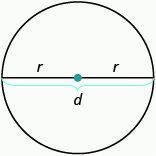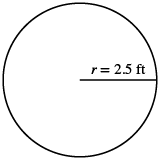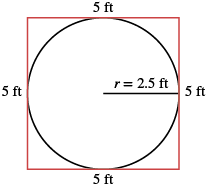## Using the Properties of Circles to Solve Problems

### Learning Outcomes

• Find the circumference and area of a circular object given its radius or diameter
• Calculate the diameter or radius of a circular object given its circumference

In a previous section, we learned the properties of circles. We’ll show them here again to refer to as we use them to solve applications.

### Properties of Circles• $r$ is the length of the radius
• $d$ is the length of the diameter
• $d=2r$
• Circumference is the perimeter of a circle. The formula for circumference is $C=2\pi r$
• The formula for area of a circle is $A=\pi {r}^{2}$

Remember that we approximate $\pi$ with $3.14$ or $\Large\frac{22}{7}$ depending on whether the radius of the circle is given as a decimal or a fraction. If you use the $\pi$ key on your calculator to do the calculations in this section, your answers will be slightly different from the answers shown. That is because the $\pi$ key uses more than two decimal places.

### example

A circular sandbox has a radius of $2.5$ feet. Find 1. the circumference and 2. the area of the sandbox.

Solution

 1. Step 1. Read the problem. Draw the figure and label it with the given information.Step 2. Identify what you are looking for. The circumference of the circle Step 3. Name. Choose a variable to represent it. Let c = circumference of the circle Step 4. Translate. Write the appropriate formula Substitute $C=2\pi r$ $C=2\pi \left(2.5\right)$ Step 5. Solve the equation. $C\approx 2\left(3.14\right)\left(2.5\right)$ $C\approx 15\text{ft}$ Step 6. Check. Does this answer make sense? Yes. If we draw a square around the circle, its sides would be $5$ ft (twice the radius), so its perimeter would be $20$ ft. This is slightly more than the circle’s circumference, $15.7$ ft.Step 7. Answer the question. The circumference of the sandbox is $15.7$ feet.
 2. Step 1. Read the problem. Draw the figure and label it with the given information.Step 2. Identify what you are looking for. The area of the circle Step 3. Name. Choose a variable to represent it. Let A = the area of the circle Step 4. Translate. Write the appropriate formula Substitute $A=\pi {r}^{2}$ $A=\pi{\left(2.5\right)}^{2}$ Step 5. Solve the equation. $A\approx \left(3.14\right){\left(2.5\right)}^{2}$ $A\approx 19.625\text{sq. ft}$ Step 6. Check. Yes. If we draw a square around the circle, its sides would be $5$ ft, as shown in part ⓐ. So the area of the square would be $25$ sq. ft. This is slightly more than the circle’s area, $19.625$ sq. ft. Step 7. Answer the question. The area of the circle is $19.625$ square feet.

### try it

in the following video we show another example of how to find the area of a circle.

We usually see the formula for circumference in terms of the radius $r$ of the circle:

$C=2\pi r$

But since the diameter of a circle is two times the radius, we could write the formula for the circumference in terms $\text{of }d$.

$\begin{array}{cccc}& & & C=2\pi r\hfill \\ \text{Using the commutative property, we get}\hfill & & & C=\pi \cdot 2r\hfill \\ \text{Then substituting }d=2r\hfill & & & C=\pi \cdot d\hfill \\ \text{So}\hfill & & & C=\pi d\hfill \end{array}$

We will use this form of the circumference when we’re given the length of the diameter instead of the radius.

### example

A circular table has a diameter of four feet. What is the circumference of the table?

### try it

In the next video we show two more examples of how to find the circumference of a circle given its diameter, or its radius.

### example

Find the diameter of a circle with a circumference of $47.1$ centimeters.

## Contribute!

Did you have an idea for improving this content? We’d love your input.